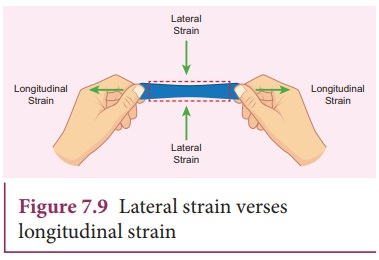Home | | Physics 11th std | PoissonŌĆÖs ratio

# PoissonŌĆÖs ratio

Suppose we stretch a wire, its length increases (elongation) but its diameter decreases (contraction).

PoissonŌĆÖs ratioSuppose we stretch a wire, its length increases (elongation) but its diameter decreases (contraction). Similarly, when we stretch a rubber band (elongation), it becomes noticeably thinner (contraction). That is, deformation of the material in one direction produces deformation in another direction. To quantify this, French Physicist S.D. Poisson proposed a ratio, known as PoissonŌĆÖs ratio, which is defined as the ratio of relative contraction (lateral strain) to relative expansion (longitudinal strain). It is denoted by the symbol ┬Ą.For a wire of length L with diameter D, due to applied force, wire stretches and hence, increase in length be l and decrease in diameter be d, thenNegative sign indicates the elongation along longitudinal and contraction along lateral dimension. Further, notice that it is the ratio between quantities of the same dimension. So, PoissonŌĆÖs ratio has no unit and no dimension (dimensionless number). The PoissonŌĆÖs ratio values of some of the materials are listed in Table 7.2.Tags : Properties of Matter , 11th Physics : UNIT 7 : Properties of Matter
Study Material, Lecturing Notes, Assignment, Reference, Wiki description explanation, brief detail
11th Physics : UNIT 7 : Properties of Matter : PoissonŌĆÖs ratio | Properties of Matter